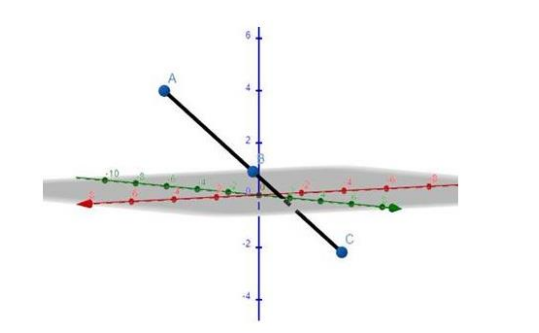# Show that the following points are collinear :

Question:

Show that the following points are collinear :

$P(3,-2,4), Q(1,1,1)$ and $R(-1,4,2)$

Solution:

To prove: the 3 points are collinear

Formula: The distance between two points $\left(x_{1}, y_{1}, z_{1}\right)$ and $\left(x_{2}, y_{2}, z_{2}\right)$ is given by

$\mathrm{D}=\sqrt{\left(x_{2}-x_{1}\right)^{2}+\left(y_{2}-y_{1}\right)^{2}+\left(z_{2}-z_{1}\right)^{2}}$

Here, Vertices should be $R(-1,4,-2)$

The solution according is

$\left(x_{1}, y_{1}, z_{1}\right)=(3,-2,4)$

$\left(x_{2}, y_{2}, z_{2}\right)=(1,1,1)$

$\left(x_{3}, y_{3}, z_{3}\right)=(-1,4,-2)$

Length $\mathrm{PQ}=\sqrt{\left(x_{2}-x_{1}\right)^{2}+\left(y_{2}-y_{1}\right)^{2}+\left(z_{2}-z_{1}\right)^{2}}$

$=\sqrt{(1-3)^{2}+(1+2)^{2}+(1-4)^{2}}$

$=\sqrt{(-2)^{2}+(3)^{2}+(-3)^{2}}$

$=\sqrt{4+9+9}$

$=\sqrt{22}$

Length $\mathrm{QR}=\sqrt{\left(x_{3}-x_{2}\right)^{2}+\left(y_{3}-y_{2}\right)^{2}+\left(z_{3}-z_{2}\right)^{2}}$

$=\sqrt{(-1-1)^{2}+(4-1)^{2}+(-2-1)^{2}}$

$=\sqrt{(-2)^{2}+(3)^{2}+(-3)^{2}}$

$=\sqrt{4+9+9}$

$=\sqrt{22}$

Length PR $=\sqrt{\left(x_{3}-x_{1}\right)^{2}+\left(y_{3}-y_{1}\right)^{2}+\left(z_{3}-z_{1}\right)^{2}}$

$=\sqrt{(-1-3)^{2}+(4+2)^{2}+(-2-4)^{2}}$

$=\sqrt{(-4)^{2}+(6)^{2}+(-6)^{2}}$

$=\sqrt{16+36+36}$

$=\sqrt{88}=2 \sqrt{22}$

$P Q+Q R=\sqrt{22}+\sqrt{22}=2 \sqrt{22}=P R$

Therefore $P, Q, R$ are collinear.